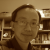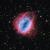# Tetrahedron## Another Tetrahedron

Thanks to Knoblauch who created the Tetrahedron blog in VRMath2 Community. She demonstrated two methods to create a tetrahedron. Here I present another way to construct a tetrahedron using VRMath2 Editor. This is a generic way utilising the dihedral angles in 3D shapes. By this, many of the classical geometric solids such as those listed in this challenge can be easily created.## Methane Molecule

This is my first and simple construction of a molecule. I chose to do Methane (CH4), one atom of carbon and four atoms of hydrogen, because it takes the shape of a tetrahedron. In this blog, I used a different but simple method to construct this tetrahedron shape. There is a special angle in tetrahedron. With this special angle, we can easily construct a tetrahedron.## Tetrahedron

The tetrahedron has the smallest number of faces in the five Platonic Solids, having only 4 faces. And in fact, four faces are the minimium requirement for a polyhedron. Other features of tetrahedron includes:

• Each face is an equilaterial triangle
• Each vertex is the meeting of 3 faces